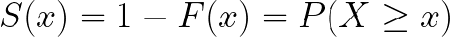top of page
Search

# Methods in Reliability

Rafael Schwarzenegger

School of Management ScienceBelow, I will describe concepts in reliability which the reader might find interesting to think about and realise. In reliability, we focus on the probability of a system to operate without failure over a given period of time.

Positioning of Reliability

From a philosophical standpoint, I would argue that reliability is related or linked to actuarial sciences. The interest or the estimation of the probability of failure over time of an entity is shared by both. In life insurance, we are usingmortality tables to calculated relevant probabilities. For lifetime analysis, we might opt for a parametric or non-parametric option. From a moratility table are insurances calculating the death rate within a specific population. It shows the population from years 0 at each year. A parametric method is one which is based on estimating the parameter of the statistical distribution from which the assumed sample originates. A non-parametric method does not use any estimated parameters for its calculations.

The reliability of products is closely linked to the study of their durability and survival analysis. Survival analysis is a collection of statistical methods, with the variable of interest being the time until an event occurs.

The area of applied statistics in industry encompasses various branches. As one of the most used, quality control should be mentioned and the use of t-tests. These can be used, as well as many other tests, to address whether a sample meets the assumed quality or characteristics. A t-test is the most common statistical test, where we test whether a sample originates from the Gaussian (also known as normal) distribution with assumed mean and unknown, but estimated variance.

A common feature in lifetime data is the presence of censoring. Lifetime data are the lifetimes of individuals or mechanical systems – their ages. It describes the situation when we miss observing a part of the system’s lifetime which results, most commonly, in having to end the experiment prematurely, due to time constraints, without having observed the failure of all components. Therefore, we do assume that these components would last some time longer. The methods incorporate the presence of censoring and make use of this information.

Companies deal with reliability for example in maintenance problems. Maintenance problems encompass the question “When is the right time to change parts of the equipment?,” leading to an optimization problem. There are some financial burdens connected with performing checks of the system condition and changing equipment. However, to fall out of the functionality of the entire system could be costly and worse.

Definitions

Continuing with the basic definitions, let us describe the distribution function as F(x)=P(X<x) describing a random variable (e.g., wheel bearing) X in the way of addressing the probability that the time to failure is smaller than x.

The survival function S(x) is the complement to the distribution function S(x)=1-F(x).

We call the survival function also the reliability function. It describes the probability that a random variable lasts at least until time x.Fig. 1 Survival Function

A special function that is introduced in reliability is the hazard function. It links to the survival function in this way:Fig. 2 Hazard Function

For a system, the hazard function is forming a bathtub curve (as described in Karpíšek, 2005; Schwarzenegger, 2017):Fig. 3 Bathtub Curve

One of possible bathtub curves is depicted above. It consists of 3 stages, namely infant mortality, useful life and wear out life.

If systems are analysed and we focus on finding the weakest link in a chain of components, we will be using fault trees. Illustrating with a simple example of a system of 2 light bulbs connected with electrified wire, if we have a series setup as in figure 4, the reliability of the system will be calculated as the product of the components R_1*R_2. Running parallel to this, the probability of failure of the system is the sum of the component failure probabilities F_1+F_2. To prove, R= R_1*R_2= (1-F_1) *(1-F_2). For a parallel system in figure B, the reliability of the system will be calculated as the sum of the underlying component reliabilities R_1+R_2 (further detailshere). Intuitively, a redundant system leads to more reliability. Therefore, a sum will produce a higher reliability than a product of those 2 numbers.Fig 4. Two lightbulb system

In a fault tree, the series system is represented with an OR gate, and the parallel system with an AND gate. For more complex situations, we might add more advanced gates like the "voting OR gate" where only some components can fail, and the system is still working.

It usually handy from a logical standpoint to transform the logical relationships into the conjunctive AND or OR form (science direct link). So, all logical relationships, may be decomposed into AND and OR relationships. For example, A implying B is equivalent to notA "OR" B. There are much morelogical equivalences. Moreover, all gates may be transformed into a NAND (notAND) gate. This is how in computers work in informatics. One gate type would be enough with which to work.

Author retains copyright to text and images.